Python Tutorial

# Python while loop Statements

Loops stand as vital components within computer programming languages, facilitating iterative processes that are executed iteratively. They provide an efficient mechanism to repeatedly perform tasks until specific conditions are met, thereby streamlining the implementation of repetitive operations. Every loop has 3 parts:

1. Initialization
2. Condition
3. Updation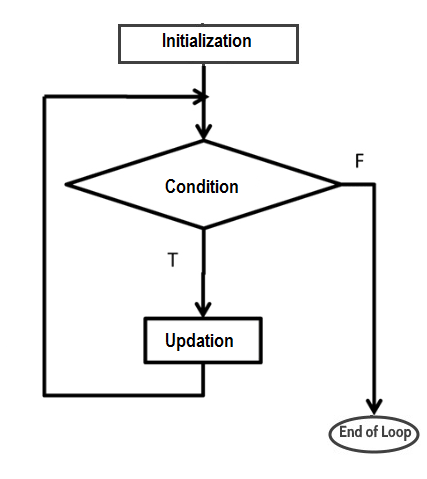## Python while loop

Syntax
while (condition) : statement(s)

The "while" loop constitutes a vital control flow construct that enables the iterative execution of code contingent upon a specified Boolean condition. Essentially, the "while" loop instructs the computer to perform a series of actions while the condition remains valid. Comprising both a condition/expression and a code block, the loop functions by evaluating the condition/expression; when deemed true, the encompassed code executes. This iterative process persists until the condition/expression transitions into a false state. This construct embodies a dynamic mechanism for accomplishing repetitive tasks within programming contexts.

initialization; while(condition) { //Code block to execute something }

To illustrate, consider the scenario where a variable 'x' is initialized to 0 and a condition of 'x <= 5' is established; this condition remains true. Conversely, when the condition shifts to 'x >= 5', it evaluates to false. Subsequent to scrutinizing the "while" loop's condition, should it validate as true, the loop's code block is executed. While within the loop, the code has the capability to modify the statements contained therein. Upon updating, the condition undergoes reevaluation. This cyclic process persists as long as the condition holds its truth; only when the condition ultimately turns false does the program break free from the loop's grasp.

example
x=0 while(x < =5): print(x) x+=1
output
0 1 2 3 4 5

In this context, the condition x <= 5 is established in the "while" statement (while(x <= 5):), with the prior declaration of 'x' and its assignment as 0 (x = 0). Consequently, the initial output is the value 0 (via print(x)), which aligns logically. Subsequently, the operation x += 1 (equivalent to x = x + 1) increments 'x' to 1. Following this update, the condition undergoes reevaluation. This cyclic process persists while the condition holds true, until its eventual falsification leads to an exit from the loop. Notably, as 'x' approaches the value of 5, the loop ceases to iterate.

## Python while loop: break and continue

Python provides two keywords that terminate a loop iteration prematurely: break and continue.

1. break leaves a loop.
2. continue jumps to the next iteration.

### break statement in Python while loop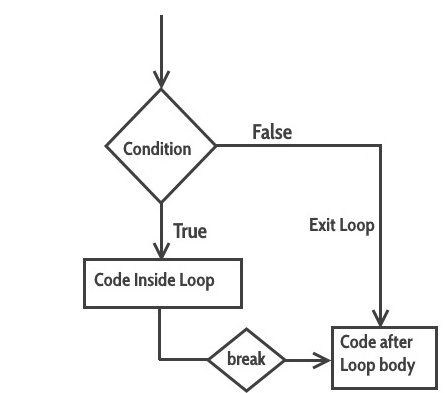Occasionally, a scenario arises where it becomes imperative to prematurely terminate a Python "while" loop before it completes its full iteration cycle. This is effectively accomplished through the utilization of a "break" statement.

example
x=10 while True: print (x) x+=2; if x>20: break print("After Break")
output
10 12 14 16 18 20 After Break

In the above example, when the condition x>20, the break statement executed and immediately terminated the while loop and the program control resumes at the next statement.

### continue statement in Python while loop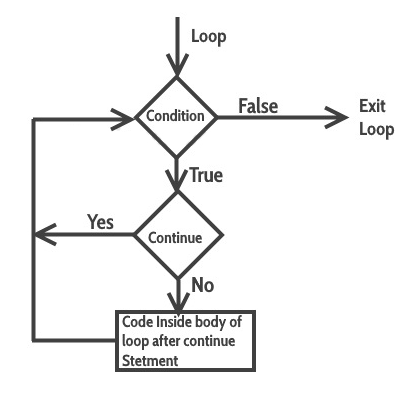The "continue" statement within a Python "while" loop serves the purpose of bypassing specific statements within the loop's body, thereby transferring control to the subsequent iteration.

example
x=0 while x < 50: x+=10 if x==30: continue print (x) print("Loop Over")
output
10 20 40 50 Loop Over

In the above example, we can see in the output the 30 is missing. It is because when the condition x==30 the loop encounter the continue statement and control go back to start of the loop.

## Else clause on Python while statement

Syntax
while (condition) : statement(s) else statement(s)

This distinctive attribute is specific to Python and distinguishes it from many other programming languages. In a Python "while" loop, the "else" clause exclusively executes when the while condition ultimately evaluates to false. However, if the loop is exited prematurely through a "break" statement or if an exception is raised, the "else" clause remains unexecuted.

example
x = 5 while (x <=10): print (x ) x = x +1 else: print(x , " Inside Else")
output
5 6 7 8 9 10 11 Inside Else

In the above example, you can see the condition is (x < =10). Up to the condition, the while block executed and the final value of x=11. Then the condition is false and the control goes to else clause of while loop and print the statement.

example
x = 11 while (x <=10): print (x ) x = x +1 else: print(x, " Inside Else")
output
11 Inside Else

Here the initial value of x=11 and condition is (x < =10). So there is no way to enter inside the while loop . So, the control directly goes to else block of while loop and print the statement.

## Nested while Loops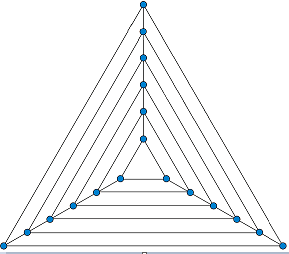A nested while loop entails a loop existing within the scope of another while loop, forming an inner loop enclosed within the body of an outer one. The mechanism involves the initiation of the outer while loop, which subsequently activates the inner while loop, allowing it to execute completely. Subsequently, the progression continues with the subsequent passes of the outer loop triggering the inner loop's execution anew. This pattern persists until the outer loop concludes. Notably, the continuity of this process can be disrupted by a "break" statement within either the inner or outer loop.

example
i = 1 while i <= 5: j = 1 while j <= i: print(j, end = '') j += 1 print() i += 1
output
1 12 123 1234 12345

## One-Line while Loops

Similar to an "if" statement, a Python "while" loop can also be condensed into a single line. In cases where the loop body consists of multiple statements, they can be separated by semicolons (;) for succinct representation.

example
x,y = 0,5 while (x<y): x +=1; print(x);
output
1 2 3 4 5

### is same as:

x = 0 y = 5 while x < y: x +=1 print(x)
output
1 2 3 4 5

## Python Infinite while Loop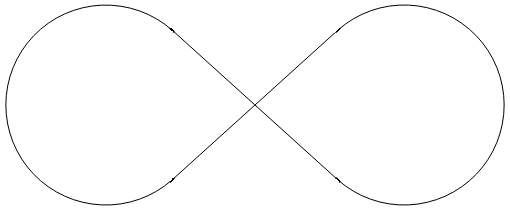True to its name, an infinite loop persists indefinitely. Within the field of computer programming, this entails the loop continuing to run until the program is deliberately terminated. An infinite loop finds utility in programs perpetually awaiting input, remaining dormant during such periods. The implementation of an infinite loop in Python employs the "while" statement, where a condition consistently evaluating to True results in an endless loop.

example
# Press Ctrl + c to exit from loop while True: print ("This is an infinite Loop")

## How to Emulate a do-while loop in Python?

Python indeed lacks a built-in "do-while" loop construct. The distinctive attributes of a "do-while" loop encompass the assurance that the loop body executes at least once, with the condition evaluated at the conclusion of the loop body's execution.

condition = True while condition: # loop body here print("Execute at least one time") condition = False
output
Execute at least one time

### Conclusion

The Python "while" loop facilitates iterative execution of a code block based on a condition. It repeatedly runs as long as the condition remains true, allowing for dynamic control flow. This construct is versatile for handling repetitive tasks and provides opportunities for nested loops and early termination through "break" and "continue" statements.